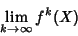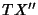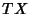## Homoclinic Point

A point where a stable and an unstable separatrix (invariant manifold) from the same fixed point or same family intersect. Therefore, the limitsandexist and are equal.Refer to the above figure. Letbe the point of intersection, withahead ofon one Manifold andahead ofof the other. The mapping of each of these pointsandmust be ahead of the mapping of,. The only way this can happen is if the Manifold loops back and crosses itself at a new homoclinic point. Another loop must be formed, withanother homoclinic point. Sinceis closer to the hyperbolic point than, the distance betweenandis less than that betweenand. Area preservation requires the Area to remain the same, so each new curve (which is closer than the previous one) must extend further. In effect, the loops become longer and thinner. The network of curves leading to a dense Area of homoclinic points is known as a homoclinic tangle or tendril. Homoclinic points appear where Chaotic regions touch in a hyperbolic Fixed Point.

A small Disk centered near a homoclinic point includes infinitely many periodic points of different periods. Poincaréshowed that if there is a single homoclinic point, there are an infinite number. More specifically, there are infinitely many homoclinic points in each small disk (Nusse and Yorke 1996).

Nusse, H. E. and Yorke, J. A. Basins of Attraction.'' Science 271, 1376-1380, 1996.